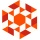Home
IT Knowledge
Inspiration
Languages
EN

# JavaScript - random element from stream of elements

9 points
Created by:Giles-Whittaker
349

In this short article, we would like to show how using JavaScript, get a random element from the stream when we don't know the number of stream elements.

Condition used to decide if the stream element should be treat as a potential randomization result is:

Practical example:

``````// ONLINE-RUNNER:browser;

const randomizeElemnt = stream => {
let result = null;
for (let i = 1; true; ++i) {
const element = stream.next();
if (element == null) {
break;
}
const number = Math.random();
if (number * i < 1.0) {
result = element;
}
}
return result;
};

// Usage example:

const createStream = count => {
let index = -1;
return {
next: () => {
index += 1;
if (index >= count) {
return null;
}
return index;
}
};
};

const stream = createStream(10); // 10 elements, next() returns next element or null
const element = randomizeElemnt(stream);

console.log(element);``````